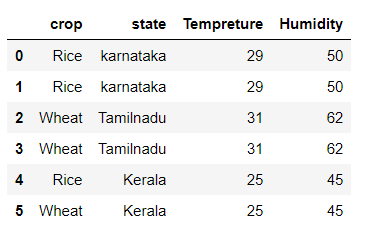Dark Mode On/Off

# Pandas DataFrame pivot() Method

In this tutorial, we will discuss and learn the Python pandas `DataFrame.pivot() `method which helps us to transform or reshape the DataFrame. When this method applied to the DataFrame, it returns the DataFrame which is reshaped and organized by the given index and column values.

The below is the syntax of the `DataFrame.pivot()` method.

### Syntax

``DataFrame.pivot(index=None, columns=None, values=None)``

### Parameters

index: It represents the str or object or a list of str, which is optional. It indicates the column to use to make new frame’s index. If this parameter is None, it uses the existing index.

columns: It represents the str or object or a list of str. It indicates the column to use to make new frame columns.

values: It represents the str, object, or a list of the previous, optional. It indicates the column or columns to use for populating new frame values. If this parameter is not specified, all remaining columns will be used and the result will have hierarchically indexed columns.

## Example 1: Reshape the DataFrame in Pandas

Let's apply the `DataFrame.pivot()` method and reshape it by specifying the index and column names. Here, in this example, we change the DataFrame's row axis to 'crop' and column axis to 'state' by passing in the index and column parameter in the `DataFrame.pivot() `method.

See the below example. The `DataFrame.pivot()` method returns the DataFrame having crops as rows and multiple columns for temperature and humidity.

``````#importing pandas as pd
import pandas as pd
#creating the DataFrame
df = df.pivot(index='crop',columns='state')
print(df)``````

## Example 2: Reshape the DataFrame using the `DataFrame.pivot()` Method

In the previous example, we have multiple columns and if we do not want multiple columns, we can specify the column name which we want in the `DataFrame.pivot() `method by passing the `values` parameter. See the below example.

The `DataFrame.pivot() `method returns the DataFrame which consists of only the Temperature column.

``````#importing pandas as pd
import pandas as pd
#creating the DataFrame
df = df.pivot(index='crop',columns='state',values='Tempreture')
print(df)``````

## Example: The `DataFrame.pivot() `method raises `ValueError`

The `DataFrame.pivot() `method raises `ValueError` while reshaping the DataFrame if there is any duplicates in the DataFrame. See the below example.

``````#importing pandas as pd
import pandas as pd
#creating the DataFrame
df = df.pivot(index='crop',columns='state',values='Tempreture')
print(df)``````ValueError: Index contains duplicate entries, cannot reshape

### Conclusion

In this tutorial, we learned the Python pandas `DataFrame.pivot()` method. We learned syntax, parameters, and solved examples by applying this method on the DataFrame and understood the method.

Want to learn coding?
Try our new interactive courses.
Over 20,000+ students enrolled.